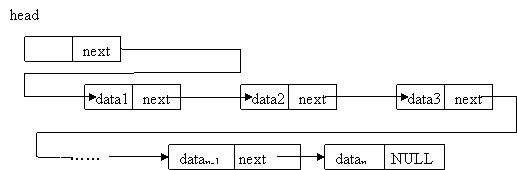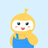• c语言链表数据存入文件读取文件
近快一年没有接触c语言了，今天学妹问我了链表数据存如文件和读取文件到链表怎么实现，现在搞开发很少用文件，都是用数据库，在这儿我还是写一下简单的文件读取链表的操作。在c语言中，创建单链表需要使用到malloc函数动态申请内存；文件的读写需要首先使用fopen函数打开文件，然后使用fscanf，fgetc, fgets，fprintf，fputc，fputs等函数读写函数，最后读写完毕要使用fclose函数关闭函数。

下面的源程序展示了关于单链表如何从文件中读取数据和往文件里存入数据。

typedef struct node {

    int data;

    struct node *next;       

}node;

 

 

//从文件中读取数据存入链表 

node *createlink()

{

    node *head =(node*)malloc(sizeof(node));

    int t;

    node *p;

    node *q;

    p=q=head;

    FILE * r= fopen("input.txt","r");

    if(r==NULL)

    {

        printf("打开文件失败!");

        return NULL; 

    }

    

    while(fscanf(r,"%d",&t)!=EOF)

    {  

       q= (node*)malloc(sizeof(node));

       q->data=t;

       p->next=q;

       p=q;

    }

    p->next=NULL;

    return head;

}

 

//输出链表到屏幕和文件output.txt 

void outlink(node *head)

{

   node *p=head->next;

   FILE *w =fopen("output.txt","w");

   if(w==NULL)

   {

       printf("打开文件失败!");

       return; 

   }

   while(p)

   {

       //输出链表节点数据到屏幕 

       printf("%d ",p->data);

       //输出链表节点数据到文件output.txt 

       fprintf(w,"%d ",p->data);

       p=p->next;        

   }     

   printf("\n");

   fprintf(w,"\n");

   fclose(w);

   return;

}

 

int main()

{

    node *head;

    int n,m;

    head=createlink();

    outlink(head);

    system("pause");

    return 0;

}


展开全文• } void fread_fp(struct node* head)//读取数据 { struct node* p =head; FILE* fp = NULL; fopen_s(&fp, "dong6.dat", "wb"); if (!fp) { printf("打开文件失败！\n"); return; } else { while (feof(fp) == 0) { ...

该楼层疑似违规已被系统折叠 隐藏此楼查看此楼
#define _CRT_SECURE_NO_WARNINGS
#include
#include
#include
struct node* list();
struct Node* colour_list();
struct node* output(struct node* p);
struct node
{
char contractnumber;//合同号
char name;//产品名称
int quantity;//数量
struct Node* colour;//颜色
struct node* next;
};
struct Node
{
char colour;//颜色
int number;//数量
struct Node* next;
};
struct node* list()//申请新节点
{
struct node* p = (struct node*)malloc(sizeof(struct node));
p->next = NULL;
return p;
}
{
struct node* p = list();
return p;
}
struct Node* colour_list()//申请颜色节点
{
struct Node* p = (struct Node*)malloc(sizeof(struct Node));
p->next = NULL;
return p;
}
void fwrite()//保存数据
{ FILE* fp = NULL;
fopen_s(&fp, "dong6.dat", "wb+");
if (!fp)
{
printf("建立文件失败！\n");
return;
}
fclose(fp);
}
{
FILE* fp = NULL;
fopen_s(&fp, "dong6.dat", "ab+");
if (!fp)
{
printf("打开文件失败！\n");
return;
}
else
{
if (p == NULL)
{
printf("没有数据无法保存！\n");
return;
}
else
{
while (p)
{
struct Node* p1 = p->colour;
while (p1)
{
fwrite(p1, sizeof(p1), 1, fp);
p1 = p1->next;
}
fwrite(p, sizeof(p), 1, fp);
p = p->next;
}
}
}
fclose(fp);
}
{
FILE* fp = NULL;
fopen_s(&fp, "dong6.dat", "wb");
if (!fp)
{
printf("打开文件失败！\n");
return;
}
else
{
while (feof(fp) == 0)
{
struct node* newp = list();
struct Node* p1 = colour_list();
newp->colour= p1;
while (p->next != NULL)
{
p = p->next;
}
while (p1)
{
p1 = p1->next;
}
p->next = newp; } }
fclose(fp);
}
void input(struct node* p)
{
char c = 'c';
struct Node* p1 = colour_list();//颜色头节点
p->colour = p1;//颜色头节点赋值
printf("请输入合同号：\n");
scanf_s("%s", &p->contractnumber, 20);
printf("请输入产品名称：\n");
scanf_s("%s", &p->name, 20);
printf("请输入合同数量：\n");
scanf_s("%d", &p->quantity);
while (1)
{
struct Node* p2 = colour_list();//颜色申请新节点
while (p1->next != NULL)
{
p1 = p1->next;
}
printf("请输入颜色：\n");
scanf_s("%s", &p2->colour, 20);
printf("请输入%s色数量：\n", p2->colour);
scanf_s("%d", &p2->number); p1->next = p2;
printf("是否有其他颜色？(y/n)\n");
scanf_s(" %c", &c, 1); if (c == 'n' || c != 'y')
{
c = 'c';
break;
}
}
}
struct node* output(struct node* p)//输出单个链表
{
struct Node* p1;
printf("合同号：%s\t产品名称：%s\t数量：%d\n", p->contractnumber, p->name, p->quantity);
p1 = p->colour->next;
while (p1)
{
int i = 0;
printf("%s\t%d\t", p1->colour, p1->number);
i++;
if (i % 2 == 0)
{
printf("\n");
}
p1 = p1->next;
}
printf("\n");
return 0;
}
{
while (p)
{
output(p);
p = p->next;
}
return 0;
}
{
{
char c = 'c';
struct node* newp = list(); if (p->next != NULL)
{
while (p->next != NULL)
{
p = p->next;
}
}
input(newp);
p->next = newp;
printf("是否有其他合同？(y/n)\n");
scanf_s(" %c", &c, 1);
if (c == 'n' || c != 'y')
{
c = 'c';
break;
}
}
return p;
}int main()
{
colorinsert_w(p);
fwrite_fp(p);
output_list(p);
return 0;
}

展开全文• 本文内容是关于：C语言 链表详解，c语言链表教程。 链表(Linked list)是一种常见的基础数据结构，是一种线性表，但是并不会按线性的顺序存储数据，而是在每一个节点里存到下一个节点的指针(Pointer)。 单链表(单向...本文将结合代码详解C语言链表，单向链表的数据结构可以分为两部分：数据域和指针域，数据域存储数据，指针域指向下一个储存节点的地址，C语言详细代码及注释如下。
/*线性表的单链表存储结构*/
typedef struct LNode {
ElemType data;
struct LNode * next;
}
LNode,
/*带有头结点的单链表的基本操作(12个)*/
/* 操作结果：构造一个空的线性表L */
* L = (LinkList) malloc(sizeof(struct LNode));
/* 产生头结点，并使L指向此头结点 */
if (!*L)
/* 存储分配失败 */
exit(OVERFLOW); ( * L) - >next = NULL;
/* 指针域为空 */
}
/* 初始條件：线性表L已存在。操作结果：销毁线性表L */
while ( * L) {
q = ( * L) - >next;
free( * L); * L = q;
}
}
/* 不改变L */
{
/* 初始条件：线性表L已存在。操作结果：将L重置为空表 */
q;
p = L - >next;
/* p指向第一个结点 */
while (p)
/* 没到表尾 */
{
q = p - >next;
free(p);
p = q;
}
L - >next = NULL;
/* 头结点指针域为空 */
}
/* 初始条件：线性表L已存在。操作结果：若L为空表，则返回TRUE，否则返回FALSE */
return L - >next == NULL;
}
/* 初始条件：线性表L已存在。操作结果：返回L中数据元素个数 */
int i = 0;
LinkList p = L - >next;
/* p指向第一个结点 */
while (p)
/* 没到表尾 */
{
i++;
p = p - >next;
}
return i;
}
Status GetElem(LinkList L, int i, ElemType * e)
/* 算法2.8 */
{
/* L为带头结点的单链表的头指针。当第i个元素存在时，其值赋给e并返回OK，否则返回ERROR */
int j = 1;
/* j为计数器 */
LinkList p = L - >next;
/* p指向第一个结点 */
while (p && j < i)
/* 顺指针向后查找，直到p指向第i个元素或p为空 */
{
p = p - >next;
j++;
}
if (!p || j > i)
/* 第i个元素不存在 */
return ERROR; * e = p - >data;
/* 取第i个元素 */
return OK;
}
int LocateElem(LinkList L, ElemType e, Status( * compare)(ElemType, ElemType)) {
/* 初始条件: 线性表L已存在，compare()是数据元素判定函数(满足为1，否则为0) */
/* 操作结果: 返回L中第1个与e满足关系compare()的数据元素的位序。 */
/* 若这样的数据元素不存在，则返回值为0 */
int i = 0;
LinkList p = L - >next;
while (p) {
i++;
if (compare(p - >data, e))
/* 找到这样的数据元素 */
return i;
p = p - >next;
}
return 0;
}
Status PriorElem(LinkList L, ElemType cur_e, ElemType * pre_e) {
/* 初始条件: 线性表L已存在 */
/* 操作结果: 若cur_e是L的数据元素，且不是第一个，则用pre_e返回它的前驱， */
/* 返回OK；否则操作失败，pre_e无定义，返回INFEASIBLE */
p = L - >next;
/* p指向第一个结点 */
while (p - >next)
/* p所指结点有后继 */
{
q = p - >next;
/* q为p的后继 */
if (q - >data == cur_e) { * pre_e = p - >data;
return OK;
}
p = q;
/* p向后移 */
}
return INFEASIBLE;
}
Status NextElem(LinkList L, ElemType cur_e, ElemType * next_e) {
/* 初始条件：线性表L已存在 */
/* 操作结果：若cur_e是L的数据元素，且不是最后一个，则用next_e返回它的后继， */
/* 返回OK;否则操作失败，next_e无定义，返回INFEASIBLE */
LinkList p = L - >next;
/* p指向第一个结点 */
while (p - >next)
/* p所指结点有后继 */
{
if (p - >data == cur_e) { * next_e = p - >next - >data;
return OK;
}
p = p - >next;
}
return INFEASIBLE;
}
Status ListInsert(LinkList L, int i, ElemType e)
/* 算法2.9。不改变L */
{
/* 在带头结点的单链线性表L中第i个位置之前插入元素e */
int j = 0;
s;
while (p && j < i - 1)
/* 寻找第i-1个结点 */
{
p = p - >next;
j++;
}
if (!p || j > i - 1)
/* i小于1或者大于表长 */
return ERROR;
/* 生成新结点 */
s - >data = e;
/* 插入L中 */
s - >next = p - >next;
p - >next = s;
return OK;
}
Status ListDelete(LinkList L, int i, ElemType * e)
/* 算法2.10。不改变L */
{
/* 在带头结点的单链线性表L中，删除第i个元素，并由e返回其值 */
int j = 0;
q;
while (p - >next && j < i - 1)
/* 寻找第i个结点，并令p指向其前岖 */
{
p = p - >next;
j++;
}
if (!p - >next || j > i - 1)
/* 删除位置不合理 */
return ERROR;
q = p - >next;
/* 删除并释放结点 */
p - >next = q - >next; * e = q - >data;
free(q);
return OK;
}
void ListTraverse(LinkList L, void( * vi)(ElemType))
/* vi的形参类型为ElemType，与bo2-1.c中相应函数的形参类型ElemType&不同 */
{
/* 初始条件：线性表L已存在。操作结果：依次对L的每个数据元素调用函数vi() */
LinkList p = L - >next;
while (p) {
vi(p - >data);
p = p - >next;
}
printf("
");
}

展开全文满意答案sdfsdwsw
推荐于 2016.01.20采纳率：47%    等级：10
已帮助：1169人
|#include
#include
struct date
{
char str;
struct date *next;
};
//链表长度为len
{
struct date *tmp;
int i;
for(i = 1; i
{
}
return tmp;
}
//读文件到链表
{
if(head == NULL || fp == NULL)
{
fprintf(stderr,"null pointer");
exit(EXIT_FAILURE);
}
do
{
}
//显示链表中的内容
{
{
fprintf(stderr,"null pointer");
exit(EXIT_FAILURE);
}
do
{
}
int main()
{
FILE *fp;
int len; //链表长度
scanf("%d",&len);
fp = fopen("a.txt","r");
exit(EXIT_SUCCESS);
}
01分享举报

展开全文• 为什么输入数据后再读取链表,链表最后一个节点的数据总是会重复输出两次代码:#include#include#define LEN sizeof(struct student)struct student{char name;long num;float chinese;float math;float english;...
• fp = fopen("storehouse.txt","w"); fwrite(); fclose(fp); int x; int n;//初次输入的大小 Node *L; if(!(L=(Node *)malloc(sizeof(Node)))) //分配空间 { printf("\n"); exit(1); } printf("欢迎...c 数据
• 如何把文件中的链表读取出来 像 typedef struct m { int a； char b; struct m *next; }M; M *p,*head,*t; 读出来之后 建立head是头的链表 文件是"D:\aaa" 存入时是 p=head; while(p !=NULL) {...
• /*创建链表*/ student *delete(student *h); /*删除记录*/ void print(student *h); /* 显示所有记录*/ void search(student *h); /*查找*/ void save(student *h); /*保存*/ student *load(); /*读入记录*/ void ...
• 当前位置:我的异常网» C语言»链表透过文件读写计算两个多项式之积链表透过文件读写计算两个多项式之积www.myexceptions.net网友分享于：2014-03-24浏览：10次链表通过文件读写计算两个多项式之积写了这个程序，...
• C语言结合文件操作的链表实例一、 功能设计一个学生成绩管理系统，要求：录入学生成绩查询学生成绩删除学生成绩修改学生成绩二、 ADT接口/* 初始化一个链表 */void InitializeList(List * plist);/* 确定链表是否为...
• c语言暂时更新完毕 链表实现 链表改进及其函数 补充知识volatile关键字 链表实现 #include <stdio.h> #include "node.h" #include <stdlib.h> //node头文件内容： //typedef struct _node{ // ...指针
• 链表的写入文件 /*====================存储彩票信息========================*/ /*功能：将彩票的全部数据写入文件 *参数：彩票数据链表 *返回值：布尔类型，读出成功返回TRUE，否则返回FALSE */ boolean ...C
• 功能：添加，删除，查找，全部输出。 添加时自动保存到文件， 查找、全部输出时从文件读取； 复制粘贴即可运行！绝对好用
• nmea-0183协议解析C语言链表实现，读取一个GPStxt对其中的nmea协议进行解析筛选
• #include #include #define N 10 typedef struct { char work_ID; char name; char phone_nu; }student; int main(int argc, char *argv[]) { student st[N];
• C语言利用文件系统实现简单的用户登录和注册功能 版本二：利用链表 此版本使用的链表，第一个版本使用的是数组 数组版本连接 这里我使用的线性链表，一定要注意在判断语句或赋值语句中不可将...
• //读取文件时跳过第一行的表头 while (1) { S studat; if (fscanf(fp, "%s\t%d\t%d\t%d\t%d\t%d\t%d\n", studat.name, &studat.num, &studat.age, &studat.math, &studat.english, &studat.physics, &studat....单链表
•数据结构 单链表
• 一 需求分析企业员工管理系统主要是针对企业员工的基本信息进行增、删、改、查的相关操作，以便用户使用本管理系统时可以快速对企业员工的信息进行管理。主要实现以下功能：用户首次使用本系统时进行密码设置和初始...
• 一.什么是链表 ...但是链表失去了数组随机读取的优点，同时链表由于增加了结点的指针域，空间开销比较大。 二.如何建立一个链表 首节点：存放第一个有效数据的节点，首节点没有前驱节点 头节点：在
• 介绍了链表与数组的应用区别与比较，并详细给出了链表使用的一些常用操作函数。数据结构 单链表 算法
• 想要实现c语言数据从链表写入到文件和从文件读取链表这个功能必须 先得了解一些关于文件操作的一些函数 fopen() fclose() fprintf() fscanf() fread() fwrite() 这些都会再我的另外一篇博客中提到 这个代码仅仅是...
• C语言链表求解多项式乘法问题 解决多项式乘法问题最容易想到的就是将乘法运算转换为加法运算。从p1的第一项开始，依此乘以p2的每一项，合并同类项后放入结果多项式中。然而项数太多时，合并同类项的过程过于复杂……...算法 数据结构
• ## C语言实现链表与文件的存取

千次阅读 多人点赞 2020-10-04 11:04:07
作者：柠檬i，学习C时长两个月半的个人练习生 第一次写文章，难免有些不足，请多多包涵。 本程序主要功能是建立链表，然后把链表数据存储到文件中，然后把文件数据存储... 参考文章： c语言链表数据存入文件读取文件
• 目录 (一)需求分析： 3 (二)概要设计： 3 ...10）实现链表文件读取的功能函数 10 11）实现链表文件存储的功能函数。 11 12）实现菜单选择的功能函数。 11 (四)系统演示及使用说明 12 1）.菜单选项 12 2
• 已有a、b两个链表，每个链表中的结点包括学号、成绩。要求把两个链表合并，按学号升序排列。 输入： 第一行，a、b两个链表元素的数量N、M,用空格隔开。 接下来N行是a的数据 然后M行是b的数据 每行数据由学号和......

# c语言链表读取txt文件c语言 订阅